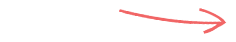# What is the answer to inflation and investment performance? – The number 70

3 min Read Published: 10 Feb 2010So what does inflation mean to people? In simple terms inflation is a measure of the increase in prices. So if inflation is high prices will rise quickly and your hard earned cash won’t go as far. If it is negative (deflation) then prices are dropping. Neither scenario is good for the economy in the long term.

But what is high and what is low? As a guide anything not around 2% is deemed bad by the Chancellor. But the answer to the question ‘what does inflation really mean to you’ is………….70!

A simple bit of mathematics (bear with me) helps make an abstract idea such as inflation a little more tangible. Put simply, if you want to know roughly how long it will take for the real value of your money to halve, simply divide the number 70 by the current rate of inflation.

So if the Retail Price Index (RPI), which is an annual measure of inflation, is say 5%. Then it will take 14 years for your money to halve in value. (70/5 = 14). Therefore you can see if inflation got out of control, say 10%, then in 7 years the buying power of your pound coin will halve.

If this simple bit of mathematics is not enough for you then this can be used to give you are more accurate idea of how inflation affects you personally.

But what else can the number 70 do?

If you want to know how long it will roughly take for your investments to double in value given a certain rate of return you again just divide the rate into the number 70.

So, if you get an invest return of 7% per annum then it will take 10 years for your money to double in size. Obviously this ignores inflation. If inflation was running at 10% as well, you would have twice as much money in nominal terms in 10 years time but the purchasing power will have remained unchanged. This is why inflation is relevant to everyone

So there you have it, if you want to know what inflation or investment returns mean in real terms then the answer is 70.

Is it true that the number 70 is the Answer to the Ultimate Question of Life, the Universe, and Everything?

No that's the number 42.

(Note - Before someone points out that the number 72, rather than 70, is often used when looking at investment returns, this is true. But the number 72 is less accurate than the number 70 when it comes to the effects of inflation. Given that this is a rough estimate it’s easier to use just one number for both. In any event, when you start using interest rates as high as 20% the accuracy starts to go out of the window).

### Looking for a financial adviser near you?

Do you need financial advice? An independent financial adviser can show you how to make the most1.A Schleisman says: